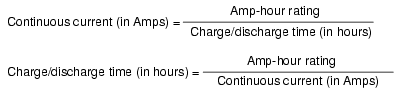# Why Battery rated in Ah (Ampere hour) and not in VA

0
835

## Why Battery rated in Ah (Ampere hour) and not in VA

• Battery stores charge in the form of chemical energy and then converts it into electrical energy to utilize for a specific time.
• The amount of available charge is the capacity of a cell or battery which may be expressed in Ah (Ampere-hour).
• Moreover, in a charged battery, the numbers of molecules are limited to create a flow of electron in electric circuits, so, there must be a limited number of electrons in a cell/battery which they motivate through a circuit to fully discharge.
• Now we have the option to rate the battery capacity in Number of flowing electrons for a specific time, but, it would be a headache, because there are a vast number of electrons in it.
• So we have another option (1C (Coulomb) = 6.25 x 10ˆ18 electrons, or 6,250,000,000,000,000,000 electrons.
• In addition, 1A (Ampere) = 1 coulomb of electrons per second and,
1h = 3600 Seconds
Therefore;
1Ah = (1A) x (3600s) = (C/s) x (3600s) = 3600 C.
∴ A (1 Ampere) = 1 Coulomb per second = C/s• The amp-hour is a unit of battery energy capacity, equal to the amount of continuous current multiplied by the discharge time, that a battery can supply before exhausting its internal store of chemical energy.• A battery with a capacity of 1 amp-hour should be able to continuously supply a current of 1 amp to a load for exactly 1 hour, or 2 amps for 1/2 hour, or 1/3 amp for 3 hours, etc., before becoming completely discharged.
• In an ideal battery, this relationship between continuous current and discharge time is stable and absolute, but real batteries don’t behave exactly as this simple linear formula would indicate.
• Therefore, when amp-hour capacity is given for a battery, it is specified at either a given current, given time, or assumed to be rated for a time period of 8 hours.

Approximate amp-hour capacities of some common batteries are given here:

• Typical automotive battery: 70 amp-hours @ 3.5 A (secondary cell)
• D-size carbon-zinc battery: 4.5 amp-hours @ 100 mA (primary cell)
• 9 volt carbon-zinc battery: 400 milliamp-hours @ 8 mA (primary cell)